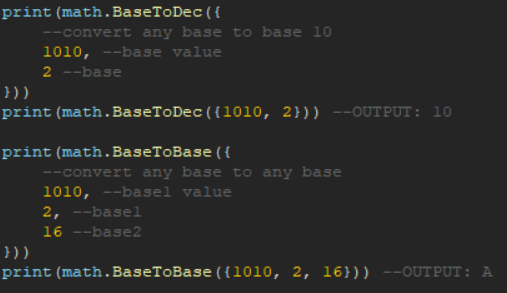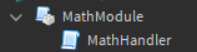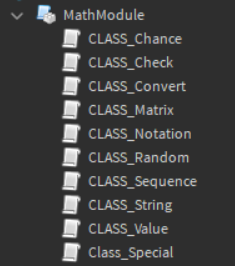# MathModule V3 -- make math easier(NEW CLASS TYPES)

MathModule V1

So what exactly does MathModule do so far?
MathModule has a total of 14 functions within it so lets get started, I will explain every single one.
When you put MathModule into your game you will get a script with its parent being MathModule, in the script you will find everything you need to get started.

line 1 through 46 you will find how the functions are called, all you need to know now is how to use all 14 of them.As you can see I explained how all of them work on the script, this is how it should look like when you open that script up.
I would love feedback on the module and I am always up to adding more math functions later for a V2.

MathModule V2

As you know V2 has released, which means even more functions.
This module has a total of 7 values and 28 total functions, which is twice the functions from V1.
So with that out of the way lets get startedThis is what you should see when you get the module.
If you look inside the Math Handler script you will see every single function and value displayed.

``````local math = require(script.Parent)
``````

As you can see to require the script you just need to add that line and change “script.Parent” to where the module is located.
Now lets get into all the functions and values.

``````print(math.EulersNumber) --OUTPUT: 2.718281828459046
print(math.EulersConstant) --OUTPUT: 0.577215664901
print(math.GammaCoeff) --OUTPUT: -0.65587807152056
print(math.GammaQui) --OUTPUT: 0.16653861138228
print(math.GammaSet) --OUTPUT: -0.042197734555571
print(math.GoldenRatio) --OUTPUT: 1.6180339887499
``````

These are the 7 values for the module.
Now lets get into the functions

``````print(math.Mean({
--find the mean out of every number in the table
1,
2,
3,
4,
5
}))
print(math.Mean({1, 2, 3, 4, 5})) --OUTPUT: 3

print(math.Median({
--find the median out of every number in the table
1,
2,
3,
4,
5
}))
print(math.Median({1, 2, 3, 4, 5})) --OUTPUT: 3

print(math.Mode({
--find the mode out of every number in the table
1,
2,
3,
4,
5
}))
print(math.Mode({1, 2, 3, 4, 5})) --OUTPUT: {...}Repeat amount

print(math.Range({
--find the range out of every number in the table
1,
2,
3,
4,
5
}))
print(math.Range({1, 2, 3, 4, 5})) --OUTPUT: 4

print(math.StandardD({
--find the Standerd Deviation out of every number in the table
1,
2,
3,
4,
5
}))
print(math.StandardD({1, 2, 3, 4, 5})) --OUTPUT: 1.4142135623731

print(math.Fac(
--find the factorial for the number given
5
))
print(math.Fac(5)) --OUTPUT: 120

--create a certain amount of numbers that add up to a certain number
--these numbers will be between the max and min variables
1, --min
5, --max
20, --final number
5 --amount of numbers
}))
print(math.RandomAdd({1, 5, 20, 5})) --OUTPUT: {...}

print(math.RandomMulti({
--create a certain amount of numbers that multiply up to a certain number
--these numbers will be between the max and min variables
1, --min
5, --max
20, --final number
2 --amount of numbers
}))
print(math.RandomMulti({1, 5, 20, 2})) --OUTPUT: {...}

print(math.ScNotation(
--convert the number given to scientific notation
100
))
print(math.ScNotation(100)) --OUTPUT: 1 * 10^2

print(math.ENotation(
--convert the number given to e-notation
100
))
print(math.ENotation(100)) --OUTPUT: 1e+2

print(math.EnNotation(
--convert the number given to engineering notation
100
))
print(math.EnNotation(100)) --OUTPUT: 100 * 10^0

print(math.DecToBase({
--convert the base 10 given to any base
10, --base 10 value
2 --base
}))
print(math.DecToBase({10, 2})) --OUTPUT: 1010

print(math.BaseToDec({
--convert any base to base 10
1010, --base value
2 --base
}))
print(math.BaseToDec({1010, 2})) --OUTPUT: 10

print(math.BaseToBase({
--convert any base to any base
1010, --base1 value
2, --base1
16 --base2
}))
print(math.BaseToBase({1010, 2, 16})) --OUTPUT: A

print(math.NthRoot({
--find the Nth root of a number
16, --Number
4 --Root
}))
print(math.NthRoot({16, 4})) --OUTPUT: 2

print(math.PerNth({
--find the Per-Nth of a number
50, --Number
100 --Nth Value
}))
print(math.PerNth({50, 100})) --OUTPUT: 0.5 or 50%

print(math.DecToRomNum(
--convert base 10 to Roman Numerals
100 --Decimal
))
print(math.DecToRomNum(100)) --OUTPUT: C

print(math.RomNumToDec(
--convert Roman Numerals to base 10
"C" --Roman Numeral
))
print(math.RomNumToDec("C")) --OUTPUT: 100

print(math.JaroS({
--find the Jaro similarity out of the strings
--the number will be between 1 and 0
--1 is an exact match while 0 is no similarity at all
"wassup", --string1
"sup" --string2
}))
print(math.JaroS({"wassup", "sup"})) --OUTPUT: 0.83333333333333

print(math.JaroD({
--find the Jaro distance out of the strings
--the number will be between 1 and 0
--0 is an exact match while 1 is no similarity at all
"wassup", --string1
"sup" --string2
}))
print(math.JaroD({"wassup", "sup"})) --OUTPUT: 0.16666666666667

print(math.JaroWS({
--find the Jaro Winkler similarity out of the strings
--the number will be between 1 and 0
--1 is an exact match while 0 is no similarity at all
"wassup", --string1
"sup" --string2
}))
print(math.JaroWS({"wassup", "sup"})) --OUTPUT: 0.83333333333333

print(math.JaroWD({
--find the Jaro Winkler distance out of the strings
--the number will be between 1 and 0
--0 is an exact match while 1 is no similarity at all
"wassup", --string1
"sup" --string2
}))
print(math.JaroWD({"wassup", "sup"})) --OUTPUT: 0.16666666666667

print(math.LevenshteinD({
--find the Levenshtein distance out of the strings
--the bigger the number the more difference the two strings have
"wassup", --string1
"sup" --string2
}))
print(math.LevenshteinD({"wassup", "sup"})) --OUTPUT: 3

print(math.MatrixMulti({
--get the product of two matrices
{1, 2, 3},
{1, 2, 3}
},
{
{5, 6, 7},
{5, 6, 7},
{5, 6, 7}
}))
print(math.MatrixMulti({{1, 2, 3}, {1, 2, 3}}, {{5, 6, 7}, {5, 6, 7}, {5, 6, 7}})) --OUTPUT: {{...}, {...}}

print(math.DotProduct(
--get the dot product of two arrays
{1, 3, -5},
{4, -2, 1}
))
print(math.DotProduct({1, 3, -5}, {4, -2, 1})) --OUTPUT: -7

print(math.CrossProduct(
--get the cross product of two arrays
{3, 4, 5},
{4, 3, 5}
))
print(math.CrossProduct({3, 4, 5}, {4, 3, 5})) --OUTPUT: {...}

print(math.TensorProduct({
--get the tensor product of two matrices
{1, 2},
{3, 4}
},
{
{5, 6},
{7, 8}
}))
print(math.TensorProduct({{1, 2}, {3, 4}}, {{5, 6}, {7, 8}})) --OUTPUT: {{...}, {...}, {...}, {...}}

print(math.MatrixTrans({
--get the transposition of a matrix
{1, 2},
{3, 4},
{5, 6},
{7, 8}
}))
print(math.MatrixTrans({{1, 2}, {3, 4}, {5, 6}, {7, 8}})) --OUTPUT: {{...}, {...}}
``````

Those are the 28 functions, everything about thing should be explained and every function has error messages incase you enter a the wrong data, which will help you out if you need help
So what do you think needs to be dealt with in V3, any suggestions are a great helpMathModule V3

welcome everyone
I am now introducing the new and improved V3
what makes V3 better then V2?
well compared to before V2 had only 35 functions
V3 has 58 functions!
and V3 has class types which is way more organized
so what exactly are class types?
well class types are to organize your code and make it so you know what you are doing
the 10 class types are

``````Value, Chance, Sequence, String, Convert, Check, Notation, Random, Matrix, and Special
``````

ok lets get into each class and break them downthis is what you will see when you get the module in game

``````local math = require(MODULE_LOCATION)
``````

change MODULE_LOCATION to where the module is located and this would be at the top of the script
with that out the way lets talk about the classes

``````--[[
this is CLASS Value
this class will have all the values in the module
]]
print(math.Value:EulersNumber()) --OUTPUT: 2.718281828459046
print(math.Value:EulersConstant()) --OUTPUT: 0.577215664901
print(math.Value:GammaCoeff()) --OUTPUT: -0.65587807152056
print(math.Value:GammaQui()) --OUTPUT: 0.16653861138228
print(math.Value:GammaSet()) --OUTPUT: -0.042197734555571
print(math.Value:GoldenRatio()) --OUTPUT: 1.6180339887499
print(math.Value:Tau()) --OUTPUT: 6.2831853071796
print(math.Value:AperysConstant()) --OUTPUT: 1.2020569031573
``````
``````--[[
this is CLASS Chance
this class will have functions related to finding any average or any probability
]]
print(math.Chance:Mean({
--find the mean out of every number in the table
1,
2,
3,
4,
5
}))
print(math.Chance:Mean({1, 2, 3, 4, 5})) --OUTPUT: 3

print(math.Chance:Median({
--find the median out of every number in the table
1,
2,
3,
4,
5
}))
print(math.Chance:Median({1, 2, 3, 4, 5})) --OUTPUT: 3

print(math.Chance:Mode({
--find the mode out of every number in the table
1,
2,
3,
4,
5
}))
print(math.Chance:Mode({1, 2, 3, 4, 5})) --OUTPUT: {...}1

print(math.Chance:Range({
--find the range out of every number in the table
1,
2,
3,
4,
5
}))
print(math.Chance:Range({1, 2, 3, 4, 5})) --OUTPUT: 4

print(math.Chance:MidRange({
--find the midrange out of every number in the table
1,
2,
3,
4,
5
}))
print(math.Chance:MidRange({1, 2, 3, 4, 5})) --OUTPUT: 3

print(math.Chance:FirstQuartile({
--find the first quartile out of every number in the table
1,
2,
3,
4,
5
}))
print(math.Chance:FirstQuartile({1, 2, 3, 4, 5})) --OUTPUT: 1.5

print(math.Chance:ThirdQuartile({
--find the third quartile out of every number in the table
1,
2,
3,
4,
5
}))
print(math.Chance:ThirdQuartile({1, 2, 3, 4, 5})) --OUTPUT: 4.5

print(math.Chance:InterquartileRange({
--find the interquartile range out of every number in the table
1,
2,
3,
4,
5
}))
print(math.Chance:InterquartileRange({1, 2, 3, 4, 5})) --OUTPUT: 3

print(math.Chance:StandardDeviation({
--find the Standerd Deviation out of every number in the table
1,
2,
3,
4,
5
}))
print(math.Chance:StandardDeviation({1, 2, 3, 4, 5})) --OUTPUT: 1.4142135623731

print(math.Chance:ZScore({
--find the ZScores for every number in the table
1,
2,
3,
4,
5
}))
print(math.Chance:ZScore({1, 2, 3, 4, 5})) --OUTPUT: {...}

print(math.Chance:Permutation({
--find the Permutations for n and r
5, --n
3 --r
}))
print(math.Chance:Permutation({5, 3})) --OUTPUT: {...}

print(math.Chance:Combination({
--find the Combinations for n and r
5, --n
3 --r
}))
print(math.Chance:Combination({5, 3})) --OUTPUT: {...}
``````
``````--[[
this is CLASS Sequence
this class will have functions related to finding a sequence of values
]]
print(math.Sequence:ThueMorse(
--find the thue morse sequence for the number given
5
))
print(math.Sequence:ThueMorse(5)) --OUTPUT: 01101001100101101001011001101001

print(math.Sequence:Integer({
--find the integers in order between min and max
1, --min
5 --max
}))
print(math.Sequence:Integer({1, 5})) --OUTPUT: {...}

print(math.Sequence:Prime({
--find the prime numbers in order between min and max
1, --min
5 --max
}))
print(math.Sequence:Prime({1, 5})) --OUTPUT: {...}

print(math.Sequence:Unprimeable({
--find the unprimeable numbers in order between min and max
1, --min
5 --max
}))
print(math.Sequence:Unprimeable({1, 5})) --OUTPUT: {...}
``````
``````--[[
this is CLASS String
this class will have functions related to strings
]]
print(math.String:JaroSimilarity({
--find the Jaro similarity out of the strings
--the number will be between 1 and 0
--1 is an exact match while 0 is no similarity at all
"wassup", --string1
"sup" --string2
}))
print(math.String:JaroSimilarity({"wassup", "sup"})) --OUTPUT: 0.83333333333333

print(math.String:JaroDistance({
--find the Jaro distance out of the strings
--the number will be between 1 and 0
--0 is an exact match while 1 is no similarity at all
"wassup", --string1
"sup" --string2
}))
print(math.String:JaroDistance({"wassup", "sup"})) --OUTPUT: 0.16666666666667

print(math.String:JaroWinklerSimilarity({
--find the Jaro Winkler similarity out of the strings
--the number will be between 1 and 0
--1 is an exact match while 0 is no similarity at all
"wassup", --string1
"sup" --string2
}))
print(math.String:JaroWinklerSimilarity({"wassup", "sup"})) --OUTPUT: 0.83333333333333

print(math.String:JaroWinklerDistance({
--find the Jaro Winkler distance out of the strings
--the number will be between 1 and 0
--0 is an exact match while 1 is no similarity at all
"wassup", --string1
"sup" --string2
}))
print(math.String:JaroWinklerDistance({"wassup", "sup"})) --OUTPUT: 0.16666666666667

print(math.String:LevenshteinDistance({
--find the Levenshtein distance out of the strings
--the bigger the number the more difference the two strings have
"wassup", --string1
"sup" --string2
}))
print(math.String:LevenshteinDistance({"wassup", "sup"})) --OUTPUT: 3
``````
``````--[[
this is CLASS Convert
this class will have functions related to converting values
]]
print(math.Convert:DecimalToBase({
--convert the base 10 given to any base
10, --base 10 value
2 --base
}))
print(math.Convert:DecimalToBase({10, 2})) --OUTPUT: 1010

print(math.Convert:BaseToDecimal({
--convert any base to base 10
1010, --base value
2 --base
}))
print(math.Convert:BaseToDecimal({1010, 2})) --OUTPUT: 10

print(math.Convert:BaseToBase({
--convert any base to any base
1010, --base1 value
2, --base1
16 --base2
}))
print(math.Convert:BaseToBase({1010, 2, 16})) --OUTPUT: A

print(math.Convert:DecimalToRomanNumeral(
--convert base 10 to roman numerals
100 --Decimal
))
print(math.Convert:DecimalToRomanNumeral(100)) --OUTPUT: C

print(math.Convert:RomanNumeralToDecimal(
--convert roman numerals to base 10
"C" --Roman Numeral
))
print(math.Convert:RomanNumeralToDecimal("C")) --OUTPUT: 100

print(math.Convert:FahrenheitToCelsius(
--convert fahrenheit to celsius
0 --Fahrenheit
))
print(math.Convert:FahrenheitToCelsius(0)) --OUTPUT: -17.777777777778

print(math.Convert:CelsiusToFahrenheit(
--convert celsius to fahrenheit
0 --Celsius
))
print(math.Convert:CelsiusToFahrenheit(0)) --OUTPUT: 32
``````
``````--[[
this is CLASS Check
this class will have functions related to checking if a value is a certain type of value
]]
print(math.Check:Integer(
--check if the number given is an integer
5
))
print(math.Check:Integer(5)) --OUTPUT: true

print(math.Check:NonInteger(
--check if the number given is a non integer
0.5
))
print(math.Check:NonInteger(0.5)) --OUTPUT: true

print(math.Check:Prime(
--check if the number given is a prime number
2
))
print(math.Check:Prime(2)) --OUTPUT: true

print(math.Check:Unprimeable(
--check if the number given is a unprimeable number
200
))
print(math.Check:Unprimeable(200)) --OUTPUT: true
``````
``````--[[
this is CLASS Notation
this class will have functions related to changing the notation of a value
]]
print(math.Notation:Scientific(
--convert the number given to scientific notation
100
))
print(math.Notation:Scientific(100)) --OUTPUT: 1 * 10^2

print(math.Notation:E(
--convert the number given to e-notation
100
))
print(math.Notation:E(100)) --OUTPUT: 1e+2

print(math.Notation:Engineering(
--convert the number given to engineering notation
100
))
print(math.Notation:Engineering(100)) --OUTPUT: 100 * 10^0
``````
``````--[[
this is CLASS Random
this class will have functions related to anything random
]]
--create a certain amount of numbers that add up to a certain number
--these numbers will be between the max and min variables
1, --min
5, --max
20, --final number
5 --amount of numbers
}))
print(math.Random:Addition({1, 5, 20, 5})) --OUTPUT: {...}

print(math.Random:Multiplication({
--create a certain amount of numbers that multiply up to a certain number
--these numbers will be between the max and min variables
1, --min
5, --max
20, --final number
2 --amount of numbers
}))
print(math.Random:Multiplication({1, 5, 20, 2})) --OUTPUT: {...}

print(math.Random:Integer({
--generate a random integer
0, --min
5 --max
}))
print(math.Random:Integer({0, 5})) --OUTPUT: N

print(math.Random:NonInteger({
--generate a random non-integer
0, --min
5 --max
}))
print(math.Random:NonInteger({0, 5})) --OUTPUT: N
``````
``````--[[
this is CLASS Matrix
this class will have functions related to matrices
]]
print(math.Matrix:Multiplication({
--get the product of two matrices
{
{1, 2, 3},
{1, 2, 3}
},
{
{5, 6, 7},
{5, 6, 7},
{5, 6, 7}
}
}))
print(math.Matrix:Multiplication({{{1, 2, 3}, {1, 2, 3}}, {{5, 6, 7}, {5, 6, 7}, {5, 6, 7}}})) --OUTPUT: {{...}, {...}}

print(math.Matrix:DotProduct({
--get the dot product of two arrays
{1, 3, -5},
{4, -2, 1}
}))
print(math.Matrix:DotProduct({{1, 3, -5}, {4, -2, 1}})) --OUTPUT: -7

print(math.Matrix:CrossProduct({
--get the cross product of two arrays
{3, 4, 5},
{4, 3, 5}
}))
print(math.Matrix:CrossProduct({{3, 4, 5}, {4, 3, 5}})) --OUTPUT: {...}

print(math.Matrix:TensorProduct({
--get the tensor product of two matrices
{
{1, 2},
{3, 4}
},
{
{5, 6},
{7, 8}
}
}))
print(math.Matrix:TensorProduct({{{1, 2}, {3, 4}}, {{5, 6}, {7, 8}}})) --OUTPUT: {{...}, {...}, {...}, {...}}

print(math.Matrix:Transposition({
--get the transposition of a matrix
{1, 2},
{3, 4},
{5, 6},
{7, 8}
}))
print(math.Matrix:Transposition({{1, 2}, {3, 4}, {5, 6}, {7, 8}})) --OUTPUT: {{...}, {...}}

print(math.Matrix:ZigZag(
--make a zigzag matrix from a whole number
5
))
print(math.Matrix:ZigZag(5)) --OUTPUT: {{...}, {...}, {...}, {...}, {...}}
``````
``````--[[
this is CLASS Special
this class will have functions related to anything that wouldn't fit the other classes
]]
print(math.Special:Factorial(
--find the factorial for the number given
5
))
print(math.Special:Factorial(5)) --OUTPUT: 120

print(math.Special:NthRoot({
--find the Nth root of a number
16, --Number
4 --Root
}))
print(math.Special:NthRoot({16, 4})) --OUTPUT: 2

print(math.Special:PerNth({
--find the Per-Nth of a number
50, --Number
100 --Nth Value
}))
print(math.Special:PerNth({50, 100})) --OUTPUT: 0.5 or 50%
``````

everything should be explained now

34 Likes

Awesome stuff! Looks great, I’ll be sure to use it when I need it!2 Likes

wow its awesome

i actually thought of making a math module recently but you took the spot3 Likes

A lot of these things that I feel that Roblox needs. This will come in handy. Thanks!

Although I don’t know a lot of the things on the list, i’m interested to see how you handled to find the mode. I made something like this that found the Median, Mode, Mean, and Mad and had a UI that let you use commands and create tables. Although I only really made it to get out of doing my math hw it was a nice project to expand my skills.

1 Like

I simply used 2 for loops in order to get it working
had to organize it and then calculate it
surprisingly I managed to do it with very little code

That’s a nice module, though some extra features, such as math.average would be very cool to see if you’d ask me.

1 Like

im hoping to have way more features in V2

did you used my setmetatable trick? awesome

1 Like

yep
I just wish I could somehow make the start of the script shorter then 46 lines
if I can im def gonna try that for the next version

isn’t finding the mean the same as finding the average?

Well, you’re correct, but having average as an alias for mean would be cool.

1 Like

so basically a second way of calling the same function
sounds like a great idea

1 Like

With math.mean being added, math.median and math.mode could be added too, differentiating the 3 types of averages. There are cases where you might want to use the last two, such as in population or player data or statistics.

2 Likes

but I already have all those three in there whatthey were talking about how average could do the same thing as math.mean but just a different name

1 Like

Bookmarked. Looks very cool! I will probably be using this

1 Like

thank you, very hype for V2 as well

1 Like

Sorry, I thought the base math library had math.average in it and that was the debate lol. Either way, using “average” for the mean is somewhat incorrect, and the other way standardizes it between the types of averaging.

1 Like

so should I even add a math.average if mean, median, mode, and range are already there?
because that would mean finding the average is just finding one of those 4 making math.average useless

Other libraries I’ve seen in other programming languages don’t use “average” as a function due to ambiguity, and ambiguity is usually a bad thing in programming for programmers, so I think it should be left out, or if it must be there, to take an input of the average type and the table to average, which would result in less performance and be redundant.

1 Like